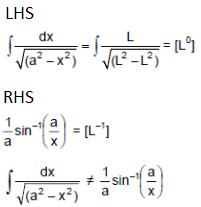# HC Verma Solutions Class 11 Chapter 1 Introduction to Physics

HC Verma Solutions Class 11 Chapter 1 Introduction to Physics consists of detailed solutions for the different topics covered in the first chapter. The solutions have been put together by our experts and are mainly aimed at providing accurate answers for Class 11 physics students.

These solutions can be incredibly beneficial for students who are looking to write JEE physics exams. In order to secure a really good rank, students should be thoroughly prepared for the variety of questions that may be asked in the examinations. The Introduction to Physics is the first chapter for class 11 and provides a basic introduction to students about the subject of physics and its branches. Referring to the HC Verma Solutions Vol 1 Chapter 1 is the simplest method to prepare effectively and be ready to solve questions related to chapters quickly and easily.

In chapter 1 students will learn many different concepts such as;

1. What is Physics?
2. Physics And Mathematics
3. Units
4. Definition Of Base Units
5. Dimensions
6. Uses Of Dimension
7. Order Of Magnitude
8. The Structure Of The World

## Class 11 Important Questions In Chapter 1

• Give the dimensions of:
(a) the volume of a cube of edge x,
(b) the volume of a sphere of radius x,
(c) the ratio of the volume of a cube of edge x to the
the volume of a sphere of radius x?
• The CGS and SI units of energy are given as erg and joule
respectively. How many ergs makeup one joule?
• How will you measure:
(a) the thickness of a sheet of paper,
(b) the distance between the earth and the moon.
• Mark the correct one. A dimensionless quantity
(a) always has a unit, (b) never has a unit,
(c) does not exist, (d) may have a unit.

## HC Verma Solutions Vol 1 Introduction to Physics Chapter 1

Question 1: Find the dimensions of

(i) Linear Momentum

(ii) Frequency and

(iii) Pressure

Solution:

(i)

Linear momentum can be written as “mv”

Dimensions of Linear momentum: mv = [MLT-1]

(ii) Frequency can be written as “1/T”, where T is time.

Dimensions of Frequency: 1/T = [M0L0T-1]

(iii) All units of pressure represent some ratio of force to area

So, dimensions of pressure: Force/Area = $\frac{[MLT^{-2}]}{[L^2]}$ = [ML-1T-2]

Question 2: Find the dimensions of

(a) Angular speed, ω

(b) Angular acceleration, α

(c) Torque, τ and

(d) Moment of Inertia, I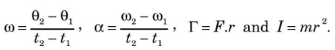Some of the equations involving these quantities are.

Solution:

(a) Dimensions of Angular speed, ω

We know, ω = θ/t

Dimensions are [M0L0T-1]

(b) Dimensions of Angular acceleration, α

We know, α = ω/t

So required dimensions are = $\frac{[M^0L^0T^{-1}]}{[T]}$ = [M0L0T-2] [using (a) result)]

(c) Dimensions of Torque, τ and

We know, τ = Fr

So, τ = [MLT-2][L] = [ML2T-2]

(d) Dimensions of Moment of Inertia, I

Here I = Mr2 = [M][L2] = [ML2T0]

Question 3: Find the dimensions of

(a) Electric Field E

(b) Magnetic field B and

(c) Magnetic permeability μ0

The relevant equations are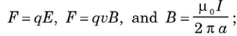where F is force, q is charge, v is speed, I is current, and a is distance.

Solution:

(a) Dimensions of Electric Field E = F/q = $\frac{[MLT^{-2}]}{[TI]}$ = [MLT-3I-1]

(b) Dimensions of Magnetic field B = F/qv = $\frac{[MLT^{-2}]}{[TI][LI^{-1}]}$ = [MT-2 I-1]

(c) Dimensions of Magnetic permeability μ0 = (Bx2 πa)/I = $\frac{[MT^{-2}I^{-1}][L]}{[I]}$ = [MLT-2 I-2]

Question 4: Find the dimensions of

(a) Electric dipole moment p and

(b) Magnetic dipole moment M.

The defining equations are p = qd and M = IA

Where d is distance, A is area, q is charge and I is current.

Solution:

a) Dimensions of Electric dipole moment p = qI = [IT] [L] = [LTI]

(b) Dimensions of Magnetic dipole moment M = IA = [I][L2] [L2I]

Question 5: Find the dimensions of Planck’s constant h from the equation E = hv where E is the energy and v is the frequency.

Solution:

Planck’s constant can be written as, h = E/v

Where E = energy and v = frequency

=> h = E/v = $\frac{[ML^2T^{-2}]}{[T^{-1}]}$ = [ML2 T-1]

Question 6: Find the dimensions of

(a) the specific heat capacity c,

(b) the coefficient of linear expansion α and

(c) the gas constant R.

Some of the equations involving these quantities are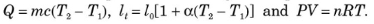Solution:

(a) Dimensions of specific heat capacity,

c = Q/mΔT = $\frac{[ML^2T^{-2}]}{[M][K]}$ = [L2 T-2K-1]

(b) Dimensions of coefficient of linear expansion,

α = $\frac{L_1-L_2}{L_0 \Delta T}=\frac{[L]}{[L][R]}=K^{-1}$

(c) Dimensions of gas constant,

R = PV/nT = $\frac{ML^{-1}T^{-2}[L^3]}{[(mol][K]}$ = [ML2 T-2K-1 (mol)-1]

Question 7: Taking force, length and time to be the fundamental quantities find the dimensions of

(a) Density

(b) Pressure

(c) Momentum and

(d) Energy

Solution:

As per given instruction, considering force, length and time to be the fundamental quantities

(a) Density = mass/volume …(1)

and, mass = Force/acceleration = (Force × time^2)/displacement.

(1)=> Density = {(Force × time^2)/displacement}/Volume

Dimensions of Density = [FL-4T2]

(b)

Pressure = F/A

Dimensions of A = [L2]

Dimensions of Pressure = [FL-2]

(c)

Momentum = mv = (force/acceleration) x velocity

= [F/LT-2] x [LT-1] = [FT]

Dimensions of Momentum [FT]

(d)

Energy = ½ mv2 = Force/acceleration x (velocity)2

$\frac{F}{[LT^{-2}]}[LT^{-1}]^2 = [FL]$

Dimensions of Energy = [FL]

Question 8: Suppose the acceleration due to gravity at a place is 10 m/s2. Find its value in cm/(minute)2.

Solution:

Given: acceleration due to gravity at a place is 10 m/s2

Convert units into cm/min2

Here, g = 10 m/sec2 = 36 x 105 cm/min2

Question 9: The average speed of a snail is 0.020 miles/hour and that of a leopard is 70 miles/hour. Convert these speeds in SI units.

Solution:

Average speed of a snail = 0.020 miles/hour (Given)

Average speed of a leopard = 70 miles/hour (Given)

In SI Units:

0.020 miles/hour = (0.02×1.6×1000)/3600 = 0.0089 m/s

[Using 1 mile = 1.6 km = 1600m]

And,

70 miles/hr = (70×1.6×1000)/3600 = 31 m/s

Question 10: The height of mercury column in a barometer in a Calcutta laboratory was recorded to be 75 cm. Calculate this pressure in SI and CGS units using the following data.

Specific gravity of mercury = 13.6

Density of water = 103 kg/m3,

gravity, g = 9.8 m/s2 at Calcutta.

Pressure = hρg in usual symbols.

Solution:

The height of mercury column in a barometer in a Calcutta laboratory was recorded to be 75 cm. (Given)

Say, h = 75 cm

Calculate pressure in SI and CGS units.

Pressure = hρg = 10 x 104 N/m2 approx

In C.G.S. units, Pressure = 10 x 105 dyne/cm2

Question 11: Express the power of a 100 watt bulb in CGS unit.

Solution:

Write power 100 watt in CGS units.

In S.I. units: 100 watt = 100 Joule/sec

In C.G.S. unit = 109 erg/sec

Question 12: The normal duration of I.Sc. Physics practical period in Indian colleges is 100 minutes. Express this period in microcenturies. 1 microcentury = 10-6 x 100 years. How many microcenturies did you sleep yesterday?

Solution:

Given: 1 microcentury = 10-6 x 100 years.

1 year = 365 x 24 x 60 min

Or 1 microcentury = 10-4 x 365 x 24 x 60 min

So, 100 min = 105/52560 = 1.9 microcentury

Question 13: The surface tension of water is 72 dyne/cm. Convert it in SI units.

Solution:

Given: surface tension of water is 72 dyne/cm

In S.I units: 72 dyne/cm = 0.072 N/m

Question 14: The kinetic energy K of a rotating body depends on its moment of inertia I and its angular speed ω. Assuming the relation to be K = kIa ωb where k is a dimensionless constant, find a and b. Moment of inertia of a sphere about its dimeter is 2/5 Mr2.

Solution:

K = kIa ωb ; where k is a dimensionless constant, K = kinetic energy and ω = angular speed

To find: a and b

Now, K = [ML2T-2]

Ia = [ML2] a and ωb = [T-1]b

=> [ML2T-2] = [ML2] a [T-1]b

[using principle of homogeneity of dimension]

Equating the dimensions, we get

2a = 2 => a = 1

-b = -2 => b = 2

Question 15: Theory of relativity reveals that mass can be converted into energy. The energy E so obtained is proportional to certain powers of mass m and the speed c of light. Guess a relation among the quantities using the method of dimensions.

Solution:

The relationship between energy, mass and speed of light is,

E ∝ MaCb

Where M = mass and C = speed of light

or E = K MaCb ……(1)

where K = constant of proportionality

Find the dimensions of (1)

[ML2T-2] = [M]a [LT-1]b

By comparing values, we have

a = 1 and b = 2

So, we have required relation is E = KMC2

Question 16: Let I = current through a conductor, R = its resistance and V = potential difference across its ends. According to Ohm’s law, product of two of these quantities equals the third. Obtain Ohm’s law from dimensional analysis. Dimensional formulae for R and V are [ML2I-2T-3] and [ML2T-3I-1] respectively.

Solution:

Given:

Dimensional formulae for R = [ML2I-2T-3] and

Dimensional formulae for V = [ML2T-3I-1]

Therefore,

[ML2T-3I-1] = [ML2I-2T-3] [I]

=> V = IR

Question 17: The frequency of vibration of a string depends on the length L between the nodes, the tension F in the string and its mass per unit length m. Guess the expression for its frequency from dimensional analysis.

Solution:

L = length, M = mass and F = Force

Here, f = KLaFbMc …(1)

Dimension of frequency, f = [T-1] or [M0L0T-1]

Dimension of length, L = [L]

Dimension of mass, M = [ML-1]

Dimension of force, F = [MLT-2]

(1)=> [M0L0T-1] = K [L]a [MLT-2]b [ML-1]c

Equating both sides, we get

b + c = 0

-c + a + b = 0

-2b = -1

Solving above three equations , we have

a = -1, b = ½ and c = -1/2

Therefore, frequency is

f = KL-1F1/2M-1/2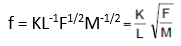Question 18: Test if the following equations are dimensionally correct: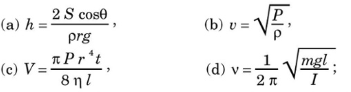where h = height, S = surface tension, ρ = density, P = pressure, V = volume, η = coefficient of viscosity, v = frequency and I = moment of inertia

Solution:

(a) Dimension of h = [L]

Dimension of S = F/I = MLT-2/L = [MT-2]

Dimension of density = M/V = [ML-3T0]

Dimension of gravity = [LT-2]

Now,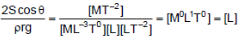Relation is correct.

(b) Let velocity = v = $\sqrt{\frac{p}{\rho}}$….(1)

Dimension of v = [LT-1]

Dimension of p = F/A = [ML-1T-2]

Dimension of ρ = m/v = [ML-3]

Substituting dimensions in (1), we have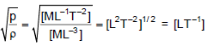Therefore, relation is correct.

(c)

Dimension of V = [L3]

Dimension of p = [ML-1T-2]

Dimension of r4 = [L4]

Dimension of t = [T]

Dimension of η = [ML-1T-1]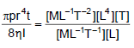Therefore, relation is correct.

(d)

Dimension of v = [T-1]

Dimension of m = [M]

Dimension of g = [LT-2]

Dimension of l = [L]

Dimension of inertia = [ML2]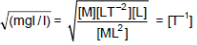Therefore, relation is correct.

Question 19: Let x and a stand for distance. Is below equation dimensionally correct?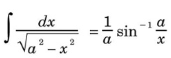Solution:

Dimensions of a = [L]

Dimensions of x = [L]